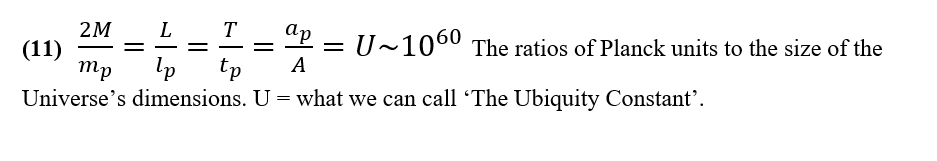## Hypersphere CosmologyAlternative to big-bang

Monday, 22 February 2021 17:01

## Equation 11Equation 11

The Dirac Large Numbers Hypothesis suggests that a huge dimensionless number, or perhaps just a few huge dimensionless numbers, seem to characterise many ratios in the observed universe that relate the quantum scale to the cosmic scale.

If the universe exists in a state of expansion, then the equality of all these ratios appears as an extraordinary coincidence at only this particular epoch of the universe and we just have the good luck to observe it now.

On the other hand, if the universe exists as a non-expanding Hypersphere, finite and unbounded in space and time, then the Large Numbers simply represent the permanent ratio between the smallest components of the universe as shown by the quantum scale and the whole universe.

The universe and the quantum realm have characteristic units of mass, length, time, energy and acceleration, the same equations govern both: -

m/l = c^2/G     t = c/l   e = mc^2   a = c^2/l

A single number underlies the ratios of the quantum scale to the cosmic scale, this number which we can call The Ubiquity Constant U has a value of the order of 10^60, or e60.

All the other ‘cosmic coincidences’ such as e20, e40, e60, e80, e120 all arise as multiples of it and reflect something fundamental about the universe at any point in time.

Amusingly the ‘vacuum catastrophe’ which arises if you mistakenly try to explain the non-existent cosmological constant in terms of the non-existent quantum vacuum energy, comes out by a factor of e120 wrong, the largest mistake cosmologists have ever admitted to.

The origin of U, the ratio of the quantum scale to the cosmological scale remains a mystery but at least it reduces all the mysterious large number coincidences to a single mystery.

Equations 12, 13, 14, and 15 show how the Ubiquity constant defines the size of quanta, the pixilation of the universe, the effective uncertainty, and the mass of stable particles in the universe.

More in this category: « Equation 12 Equation 10 »
• #### HyperSphere Cosmology +Hypersphere Cosmology. (6)    P J Carroll 28/2/2021   Abstract.
• #### Type 1A supernovae and Hypersphere Cosmology +

Lensing, Redshift and Distance for Type1A Supernovae. All data input from Perlmutter et all, https://arxiv.org/pdf/astro-ph/9812133.pdf The measured Apparent Magnitudes of
• #### Galactic Rotation Curves +

Galactic Rotation Curves. A large discrepancy exists between the rotation curves for disc galaxies expected from a classical or relativistic
• #### Equation 17 +

Equation 17 The Redshift-Distance and the Lensing equations 6 and 16 together yield a derivation for the Antipode length L
• #### Equation 16 +

Equation 16 A stereographic projection can show the effect of projecting an n-sphere into an n-dimensional space. For
• #### Equation 15 +

Equation 15 It may seem odd that whilst the ‘enhanced’ Planck length and Planck time set a lower limit for
• #### Equation 14 +

Equation 14 The universe has an information deficit in the sense that it has only one bit of information/entropy for
• #### Equation 13 +

Equation 13 The universe has an information deficit in the sense that it has only one bit of information/entropy for
• #### Equation 12 +

Equation 12 The Bekenstein-Hawking conjecture arose to explain the behaviour of entropy in a black hole. https://en.wikipedia.org/wiki/Black_hole_thermodynamics#Overview It asserts that
• #### Equation 11 +

Equation 11 The Dirac Large Numbers Hypothesis suggests that a huge dimensionless number, or perhaps just a few huge dimensionless
• #### Equation 10 +

Equation 10 Here we show the modification to Newtonian Dynamics expected in a positively curved spacetime that has a negative
• #### Equation 9 +

Equation 9 Galaxies rotate but they do not rotate in accordance with our standard ideas about gravity. A large discrepancy
• 1
• 2